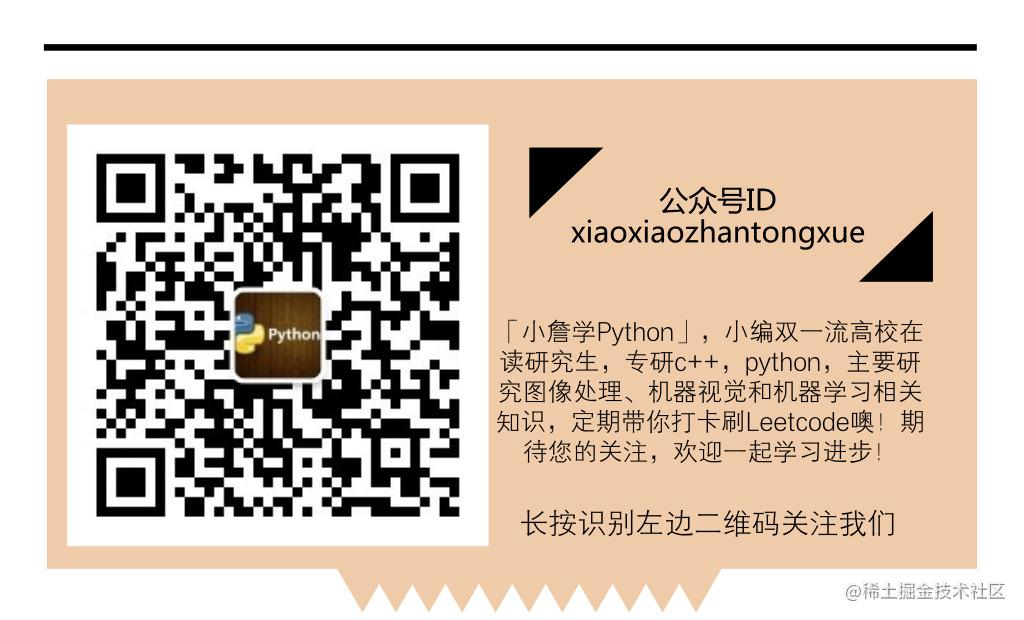# 拿什么拯救你，我的面试之——从零打卡刷Leetcode（No.003）

【欢迎关注个人微信公众号【小詹学python】】

``````Finished in 36 ms
[7, 0.6999999999999993, 8.07, 1]

``````carry = int(p.next.val / 10)  #int()强制转换为整型

No.3 Longest Substring without Repeating Characters

Given a string, find the length of the longest substring without repeating characters.

``````Given "abcabcbb", the answer is "abc", which the length is 3.
Given "bbbbb", the answer is "b", with the length of 1.
Given "pwwkew", the answer is "wke", with the length of 3. Note that the answer must be a substring, "pwke" is a subsequence and not a substring.

``````def lengthOfLongestSubstring(self, s):
"""
:type s: str
:rtype: int
"""
max_len = 0 #用这个值记录我们要返回的最长子字符串长度
#当原字符串长度为0或1的特殊情况
if (len(s) == 1 or len(s) == 0):
max_len = len(s)
#开始遍历每一个子字符串，并进行长度比较，得到最长的那个
for i in range(0,len(s)-1):
for j in range(i+1, len(s)):
if s[j] in s[i:j]:
if j-i > max_len:
right = j
left = i
#这里小詹本想返回对应子字符串的左右索引值，之后发现题目没有要求
max_len = right-left
break
elif j == len(s) - 1:
if max_len < j - i + 1:
max_len = j - i + 1
return max_len

``````def lengthOfLongestSubstring(self, s):
"""
:type s: str
:rtype: int
"""
#创建一个空字典，其存放的形式是“单字符:出现位置的索引”
indexDict = {}
#存放记录最大长度和当前循环下的长度
maxLength = currMax = 0
for i in range(len(s)):
#这里是关键，小詹看了挺久的，小伙伴们比我强，应该比较快
#这里是当s[i]没有在之前出现过，则当前长度currMax自动加一
#当出现了重复字符，则比较当前找到的子字符串长度和历史最大长度
#重点是这里i - indexDict[s[i]] - 1 的含义；代码后举例具体讲解
if s[i] in indexDict and i - indexDict[s[i]] - 1 <= currMax:
if maxLength < currMax:
maxLength = currMax
currMax = i - indexDict[s[i]] - 1
currMax = currMax + 1
indexDict[s[i]] = i
return maxLength if currMax < maxLength else currMax